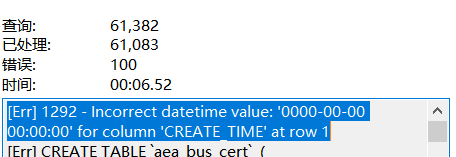TypeScript 实战技巧

## 一、常用技巧

### 1. 函数重载

``````    export function util(str: string): string    export function util(str: number): number    export function util(str: string | number): number | string {      return str;    }        const a: string = util('a');    const b: number = util(1);
``````

### 2. 捕获变量类型

``````    let a = 'abc';    let b = { x: 0, y: 1 };        type A = typeof a; // string 类型    type B = typeof b; // { x: number, y: number }
``````

### 3 . 函数绑定 `this` 上下文

``````    const obj = {      say(name: string) {        console.log('Hello,', name);      }    }        // 通过第一个参数 this 绑定当前函数上下文    function foo(this: typeof obj, str: string) {      this.say(str);    }
``````

### 4. 使用 Never

``````    // 抛出异常    function unexpected(): never {      throw Error('Unexpected');    }        function test(x: boolean): number {      if (x) {        return 1;      }      unexpected(); // 因为 never 表示永远不会发生的类型，所以这里不会要求 number 作为返回值    }        // 官方文档给的死循环的例子    function infiniteLoop(): never {      while (true) {}    }
``````

### 5. 索引签名

``````    interface A {      x: number    }    const a: A = {x: 1}        // 接口键类型为: string, 值类型为: boolean    interface B {      [key: string]: boolean    }    const b: B = {      a: true,      b: false    }        // in 表示遍历，键名称包括: 'a', 'b', 'c'，值类型为: string    type C = {      [key in 'a' | 'b' | 'c']: string    }    const c: C = {      a: '1',      b: '2',      c: '3'    }
``````

### 6. 通过索引访问类型

``````    interface A {      a: string      b: {        c: boolean      }    }        type Aa = A['a'] // string 类型    type Abc = A['b']['c'] // boolean 类型    type B = [string, number]    type B0 = B['0'] // string 类型    type B1 = B['1'] // number 类型
``````

### 7. 使用 `as`

``````    function foo(str?: string) {      return (str as string).toString();    }        const obj = {      toString() {      }    }    foo(obj as string)
``````

### 8. 条件类型

``````    // 与 JS 的三元表达式很类似，如果 T 的类型是 string, 则返回 string 类型，否则，返回 number 类型    type F<T> = T extends string ? string : number        const a: F<string> = 'abc'; // 泛型的类型是 string, 则为 string 类型    const b: F<number> = 1; // 泛型的类型是非 string, 则为 number 类型    const c: F<boolean> = 2; // 泛型的类型是非 string, 则为 number 类型
``````

## 二、泛型

### 1. 使用示例

• function

function foo(arg: T): T {      return arg;    }

• class

class Foo {      name: T          constructor(name: T) {        this.name = name;      }          getName(): T {        return this.name      }    }        const foo = new Foo(3);    const name: number = foo.getName();

• interface

interface Foo {      a: T    }        const foo: Foo = { a: 1 }type    type Foo = { a: T }    const foo: Foo = { a: false }

### 2. 泛型约束

``````    // 指定泛型的类型限制于: string | number, 其他类型将会报错    interface A<T extends string | number> {      [key: string]: T    }        const a: A<string> = {a: 'abc'}    const b: A<number> = {a: 123}
``````

### 3. 指定泛型默认类型

``````    interface B<T = number> {      [key: string]: T    }        const c: B = { a: 123 }    const d: B<string> = { a: 'abc' }
``````

## 三、TypeScript 中的一些运算符

• `?`: 表示可选的参数或属性，可以用于函数参数、接口的属性等

• `-?`: 取消可选标识（扩展：类似的还有 `-readonly` 表示取消 readonly 标识）

• `in`: 表示遍历类型

• `typeof`: 捕获变量类型，上面有例子

• `keyof`: 将对象类型生成其键的字符串或数字的集合，常与 `in` 结合使用

• `infer`: 表示推断类型，下面讲 TS 内置类型 `Parameters` 实现的时候会有具体例子

## 四、实现 TypeScript 内置类型

### 1. Record

``````    // keyof any 包含: string | number | symbol    type Record<K extends keyof any, T> = {      // 表示键类型是约束于 K (string | number | symbol) 中的一种或多种，值类型为 T      // in 表示遍历      [P in K]: T    }        const foo: Record<string, boolean> = {      a: true    }    const bar: Record<'x' | 'y', number> = {      x: 1,      y: 2    }
``````

### 2. Partial

``````    type Partial<T> = {      // 将原始类型 T 的所有属性加上 ? 修饰，变成可选的      [P in keyof T]?: T[P]    }        interface Foo {      a: string      b: number    }        const foo: Partial<Foo> = {      b: 2 // `a` 已经不是必须的了    }
``````

### 3. Required

``````    type Required<T> = {      // 将原始类型 T 的所有属性加上 -? 修饰，变成必须的      // -? 表示移出 ? 这个标识      [P in keyof T]-?: T[P]    }        interface Foo {      a: string      b?: number    }        const foo: Required<Foo> = {      a: 'abc',      b: 2 // `b` 已经变成必选的了    }
``````

``````    type Readonly<T> = {      // 将原始类型 T 的所有属性加上 readonly 修饰，属性变成只读的      readonly [P in keyof T]: T[P]    }        interface Foo {      a: string    }        const foo: Readonly<Foo> = {      a: 'abc'    }    // foo.a = 'def' // 只读，不可修改            // 此外，可以使用 -readonly 修饰，表示去掉 readonly 修饰    type Writable<T> = {      -readonly [P in keyof T]: T[P]    }        interface Bar {      readonly a: string    }        const bar: Writable<Bar> = {      a: 'abc'    }    bar.a = 'def'; // `Bar['a'] 已经去掉了 readonly 修饰，可以修改了
``````

### 5. Pick

``````    type Pick<T, K extends keyof T> = {      [P in K]: T[P]    }        interface Foo {      a: string      b: number      c: boolean    }        const foo: Pick<Foo, 'b' | 'c'> = {      b: 1,      c: false    }
``````

### 6. Exclude

``````    // 如果 T 是 U 的子类型，则返回 never, 否则返回 T    type Exclude<T, U> = T extends U ? never : T        // 'a' | 'b' | 'c' 中排除掉 'b', 只能为 'a' 或 'c'    let foo: Exclude<'a' | 'b' | 'c', 'b'> = 'a'    foo = 'c'
``````

### 7. Extract

``````    // 如果 T 是 U 的子类型，则返回 T, 否则返回 never    type Extract<T, U> = T extends U ? T : never        // 'a' | 'b' | 'c' 中提取 'b', 只能为 'b'    let foo: Extract<'a' | 'b' | 'c', 'b'> = 'b'
``````

### 8. Omit

``````    // 1. 从 T 的属性中排除掉 K，得到剩下的一个属性集合    // 2. 从 T 中选择剩下的集合    type Omit<T, K extends keyof any> = Pick<T, Exclude<keyof T, K>>        interface Foo {      a: string      b: number      c: boolean    }        // 从 Foo 中省略 'c', 接口 Foo 只剩下 'a' 和 'b'    let foo: Omit<Foo, 'c'> = {      a: 'a',      b: 1    }
``````

### 9. NonNullable

``````    // 排除掉 null | undefined    type NonNullable<T> = T extends null | undefined ? never : T        type Foo = string | null    const a: NonNullable<Foo> = 'a' // 不能赋值给 null 或 undefined
``````

### 10. Parameters

``````    // 主要说一下 infer P, 在这里表示待推断的函数参数    type Parameters<T extends (...args: any) => any> = T extends (...args: infer P) => any ? P : never        type Foo = (a: string, b: number) => void    const a: Parameters<Foo> = ['a', 1] // 元组 [string, number]
``````

### 11. ConstructorParameters

``````    // 跟 Parameters 类似    type ConstructorParameters<T extends new (...args: any) => any> = T extends new (...args: infer P) => any ? P : never        interface Foo {      new(a: string, b: number)    }        const a: ConstructorParameters<Foo> = ['a', 1] // 元组 [string, number]
``````

### 12. ReturnType

``````    // 跟 Parameters 类似    type ReturnType<T extends (...args: any) => any> = T extends (...args:any) => infer R ? R : any        type Foo = () => boolean    const a: ReturnType<Foo> = true // 返回 boolean 类型
``````

### 13. InstanceType

``````    // 跟 Parameters 类似    type InstanceType<T extends new (...args: any) => any> = T extends new (...args: any) => infer R ? R : any
``````

## 参考链接

• [TypeScript 手册参考][TypeScript]

• [深入理解 TypeScript][TypeScript 1]

• [TS 中的内置类型简述][TS]blmius
2年前
MySQL:[Err] 1292 - Incorrect datetime value: ‘0000-00-00 00:00:00‘ for column ‘CREATE_TIME‘ at row 1Jacquelyn38
2年前
2020年前端实用代码段，为你的工作保驾护航Wesley13
1年前
java将前端的json数组字符串转换为列表helloworld_34035044
1年前

​在我们用皕杰报表工具设计填报报表时，如何在新增行里自动增加id呢？能新增整数排序id吗？目前可以在新增行里自动增加id，但只能用uuid函数增加UUID编码，不能新增整数排序id。uuid函数说明：获取一个UUID，可以在填报表中用来创建数据ID语法：uuid()或uuid(sep)参数说明：sep布尔值，生成的uuid中是否包含分隔符''，缺省为Wesley13
1年前
mysql设置时区
mysql设置时区mysql\_query("SETtime\_zone'8:00'")ordie('时区设置失败，请联系管理员！');中国在东8区所以加8方法二：selectcount(user\_id)asdevice,CONVERT\_TZ(FROM\_UNIXTIME(reg\_time),'08:00','0Stella981
1年前
JS 对象数组Array 根据对象object key的值排序sort,很风骚哦Stella981
1年前
HIVE 时间操作函数Wesley13
1年前
00：Java简单了解Stella981
1年前
JavaScript常用函数
1\.字符串长度截取functioncutstr(str,len){vartemp,icount0,patrn/^\x00\xff/，strre"";for(variWesley13
1年前
MySQL部分从库上面因为大量的临时表tmp_table造成慢查询Easter79
Lv1

2.8k

5

1.2k